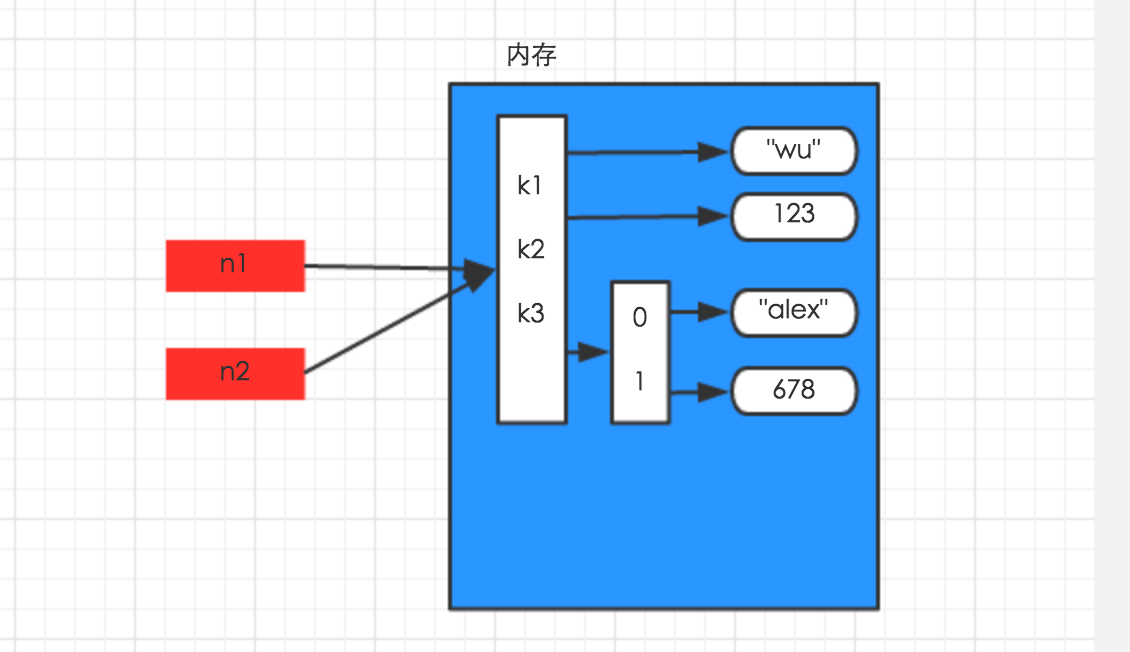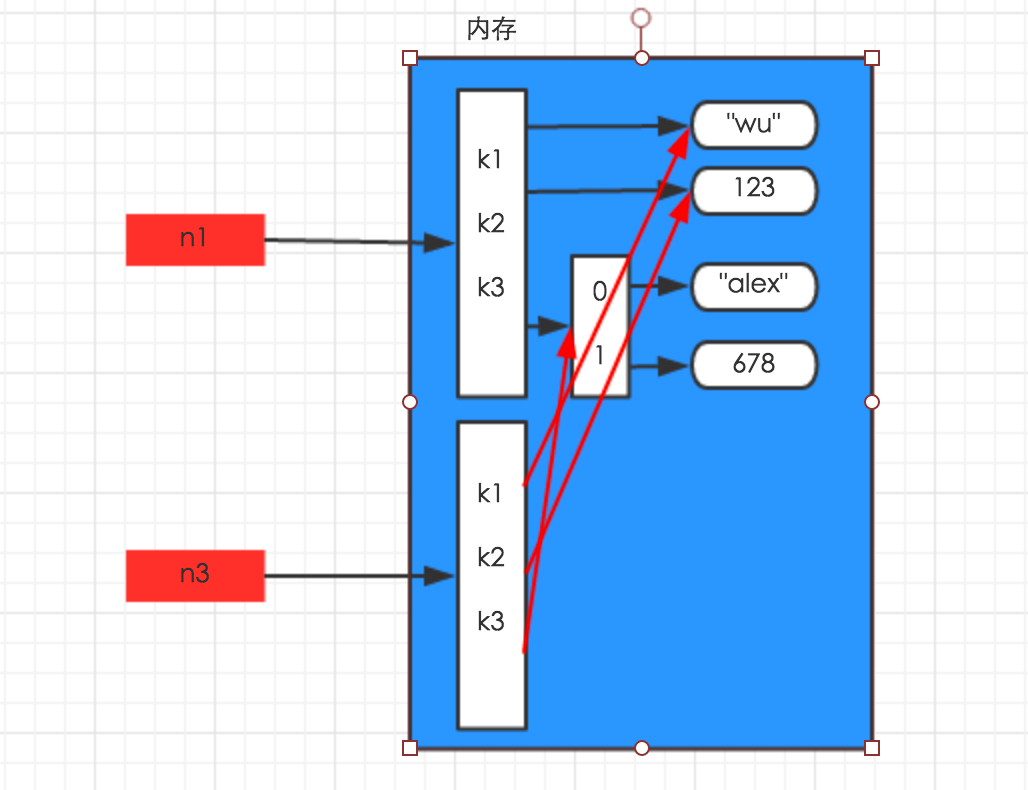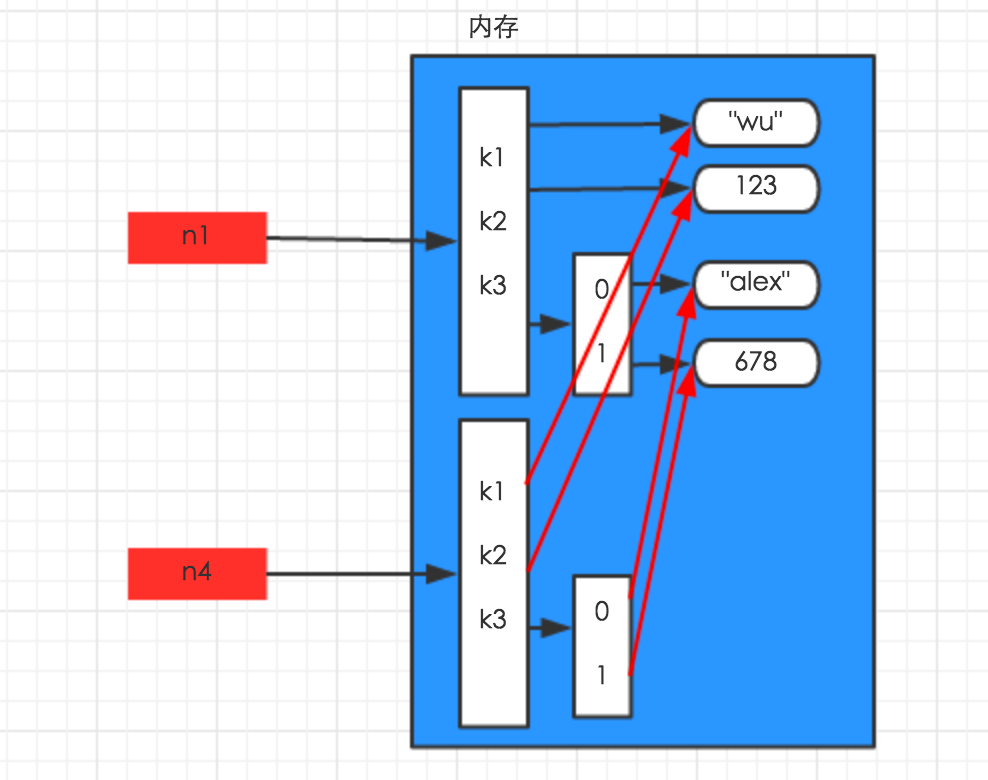+关注

# python基础题（53道题详解）

## 1、简述解释型和编译型编程语言？

### 概念：

• 编译型语言：把做好的源程序全部编译成二进制代码的可运行程序。然后，可直接运行这个程序。
• 解释型语言：把做好的源程序翻译一句，然后执行一句，直至结束！

### 区别：

• 编译型语言，执行速度快、效率高；依赖编译器、跨平台性差些。如C、C++、Delphi、Pascal，Fortran。
• 解释型语言，执行速度慢、效率低；依赖解释器、跨平台性好。如Java、Basic.

## 2、Python解释器种类以及特点

• CPython
• c语言开发的 使用最广的解释器
• IPython
• 基于cpython之上的一个交互式计时器 交互方式增强 功能和cpython一样
• PyPy
• 目标是执行效率 采用JIT技术 对python代码进行动态编译，提高执行效率
• JPython
• 运行在Java上的解释器 直接把python代码编译成Java字节码执行
• IronPython
• 运行在微软 .NET 平台上的解释器，把python编译成. NET 的字节码

## 3、python常见的PEP8规范

• 每级缩进用4个空格
• Python 3中不允许混合使用Tab和空格缩进。
• 限制所有行的最大行宽为79字符。
• 在核心Python发布的代码应该总是使用UTF-8(ASCII在Python 2)。
• 推荐绝对路径导入，因为它们通常更可读

## 4、通过代码实现如下进制转换：

```1 hex()
2 转换一个整数对象为十六进制的字符串
3
4 >>> hex(16)
5 '0x10'
6 >>> hex(18)
7 '0x12'
8 >>> hex(32)
9 '0x20'```
```1 oct()
2 转换一个整数对象为八进制的字符串
3
4 >>> oct(8)
5 '0o10'
6 >>> oct(166)
7 '0o246'```
```1 bin()
2 转换一个整数对象为二进制字符串
3
4 >>> bin(10)
5 '0b1010'
6 >>> bin(255)
7 '0b11111111'```
``` 1 chr()
2 转换一个[0, 255]之间的整数为对应的ASCII字符
3
4 >>> chr(65)
5 'A'
6 >>> chr(67)
7 'C'
8 >>> chr(90)
9 'Z'
10 >>> chr(97)
11 'a'```
```1 ord()
2 将一个ASCII字符转换为对应整数
3
4 >>> ord('A')
5 65
6 >>> ord('z')
7 122```
``` 1 16进制转10进制
2 >>> int('10', 16)
3 16
4 >>> int('0x10', 16)
5 16
6
7 8进制转10进制
8 >>> int('0o10', 8)
9 8
10 >>> int('10', 8)
11 8
12
13 2进制转10进制
14 >>> int('0b1010', 2)
15 10
16 >>> int('1010', 2)
17 10```

## 5、python递归的最大层数

``` 1 import sys
2 sys.setrecursionlimit(100000)
3
4 def foo(n):
5     print(n)
6     n += 1
7     foo(n)
8
9 if __name__ == '__main__':
10     foo(1)```

## 6、三元运算规则以及应用场景

•         三元运算符就是在赋值变量的时候，可以直接加判断，然后赋值
•         三元运算符的功能与'if....else'流程语句一致，它在一行中书写，代码非常精炼，执行效率更高
•         格式：[on_true] if [expression] else [on_false]
•         res = 值1 if 条件 else 值2

## 7、列举 Python2和Python3的区别

• print
• input
• ``` 1 问题：如何获取编码方式的信息？
2 获取目标bytes的编码方式
3 这一情况可以通过chardet模块的detect()函数来获取信息，chardet是第三方库，可以通过pip来安装
4
5 b是待检测的bytes变量
6
7 import chardet
8 print(chardet.detect(b))
9 ######output####
10  {'confidence': 1.0, 'encoding': 'ascii'}
11 1
12 2
13 3
14 4
15 5
16 confidence是指匹配程度，encoding是指可能的编码方式
17
18 获取当前环境的编码方式
19 这一情况可以使用sys模块下的getdefaultencoding()函数来获取信息
20
21 import sys
22 print(sys.getdefaultencoding())
23
24 ######## output#####
25 utf-8```
• 问题在控制台上看到的到底是什么
``` 1 写上面的东西的时候产生了一个疑问，现在已经知道Python内部存储str的方式是使用unicode字符集，但是我们在屏幕上看到的并不是unicode字符集
3 s = "你好"
4 print(s)
6 #########output#############
7 你好
13 s的 unicode 是 \u4f60\u597d
14 1
15 那么，这中间应该是进行了某种转换
16 实际上，在执行print(str)的时候，python内部执行了encoding操作，控制台拿到的其实是一个bytes变量
17 之后，控制台又根据环境内部的编码方式，将所得到的bytes内容进行decoding的操作，就显示了原先str的内容```
• 打开文件不再支持 file 方法，只能用 open
• range不再返回列表，而是一个可迭代的range对象
• 除法 / 不再是整除，而是得到浮点数，整除需要用双斜杠 //
• urllib和urllib2合并成了urllib，常用的urllib2.urlopen()变成了urllib.request.urlopen()
• 字符串及编码相关有大变动，简单来说就是原来的str变成了新的bytes，原来的unicode变成了新的str。

## 8、xrange和range的区别

python2中 xrange 用法与 range 完全相同，所不同的是生成的不是一个list对象，而是一个生成器。

## 10、列举布尔值为False的常见值

None、""、0、[]、()、{}

## 11、字符串、列表、元组、字典每个常用的5个方法（整型，浮点，字符串，布尔型，列表、元组、字典、集合、日期）

#### 字符串：

```# encoding:utf-8
__author__ = 'Fioman'
__date__ = '2018/11/19 15:10'

# 1. 去掉空格和特殊符号
name = " abcdefgeyameng  "
name1 = name.strip()  # 并不会在原来的字符串上操作,返回一个去除了两边空白的字符串
print(name1, len(name1), name, len(name))
# abcdefgeyameng 14  abcdefgeyameng   17

# 去掉左边的空格和换行符
name2 = name.lstrip()
print(name2, len(name2))# print(name2, len(name2))#

# 去掉右边的空格和换行符
name3 = name.rstrip()
print(name3, len(name3)) # abcdefgeyameng 15

# 2.字符串的搜索和替换
name.count('e')  # 查找某个字符在字符串中出现的次数
name.capitalize() # 首字母大写
name.center(100,'-') # 把字符串方中间,两边用-补齐,100表示占位多少
name.find('a') # 找到这个字符返回下标,多个时返回第一个,不存在时返回-1
name.index('a') # 找到这个字符返回下标,多个时返回第一个,不存在时报错
print(name.replace(name,'123')) # 字符串的替换
name.replace('abc','123') # 注意字符串的替换的话,不是在原来的字符串上进行替换.而是返回一个替换后的字符串.

# 3.字符串的测试和替换函数
name.startswith("abc") # 是否以abc开头
name.endswith("def") # 是否以def结尾
name.isalnum() # 是否全是字母和数字,并且至少包含一个字符
name.isalpha() # 是否全是字母,并至少包含一个字符
name.isdigit() # 是否全是数字,并且至少包含一个字符
name.isspace() # 是否全是空白字符,并且至少包含一个字符
name.islower() # 是否全是小写
name.isupper() # 是否全是大写
name.istitle() # 是否是首字母大写

# 4.字符串的分割
name.split('') # 默认按照空格进行分隔,从前往后分隔
name.rsplit() # 从后往前进行分隔

# 5.连接字符串
'.'.join(name) # 用.号将一个可迭代的序列拼接起来

name = 'geyameng'
# 6.截取字符串(切片)
name1 = name[0:3] # 第一位到第三位的字符,和range一样不包含结尾索引
name2 = name[:] # 截取全部的字符
name3 = name[6:] # 截取第6个字符到结尾
name4 = name[:-3] # 截取从开头到最后一个字符之前
name5 = name[-1] # 截取最后一个字符
name6 = name[::-1] # 创造一个与原字符串顺序相反的字符串
name7 = name[:-5:-1] # 逆序截取```

```# encoding:utf-8
__author__ = 'Fioman'
__date__ = '2018/11/19 16:26'

# 1.创建一个列表
list1 = ['1', '2', '3', '4']
list2 = list("1234")
print(list1, list2)
print(list1 == list2)
# 以上创建的两个列表是等价的,都是['1', '2', '3', '4']

# 2.添加新元素
# 末尾追加
a = [1, 2, 3, 4, 5]
a.append(6)
print(a)

# 指定位置的前面插入一个元素
a.insert(2, 100)  # 在下标为2的前面插入一个元素100
print(a)

# 扩展列表list.extend(iterable),在一个列表上追加一个列表
a.extend([10, 11, 12])
print(a)

# 3.遍历列表
# 直接遍历
for i in a:
print(i)

# 带索引的遍历列表
for index, i in enumerate(a):
print(i, index)

# 4.访问列表中的值,直接通过下标取值.list[index]
print(a)

# 从list删除元素
# List.remove() 删除方式1:参数object 如果重复元素,只会删除最靠前的.
a = [1,2,3]
a.remove(2) # 返回值是None

# List.pop()  删除方式2:pop 可选参数index,删除指定位置的元素 默认为最后一个元素
a = [1,2,3,4,5]
a.pop()
print(a)

a.pop(2)
print(a)

# 终极删除,可以删除列表或指定元素或者列表切片,list删除后无法访问
a = [1,2,3,4,5,6]
del  a
print(a) # 1, 3, 4, 5, 6]

del a[1:]
print(a) # 1

del a
# print(a) # 出错,name a is not defined

# 排序和反转代码
# reverse 反转列表
a = [1,2,3,4,5]
a.reverse()
print(a)

# sort 对列表进行排序,默认升序排列.有三个默认参数cmp = None,key = None,reverse = False

# 7.Python的列表的截取与字符串操作类型相同,如下所示
L = ['spam','Spam','SPAM!']
print(L[-1]) # ['SPAM']

# 8.Python列表操作的函数和方法
len(a)  # 列表元素的个数
max(a)  # 返回列表元素最大值
min(a)  # 返回列表元素最小值
list(tuple) #将一个可迭代对象转换为列表

# 列表常用方法总结
a.append(4)
a.count(1)
a.extend([4,5,6])
a.index(3)
a.insert(0,2)
a.remove()
a.pop()
a.reverse()
a.sort()```

```1.用一个可迭代对象生成元组
T = tuple('abc')```
```对元组进行排序

T = ('c','a','d','b')
tmp = list(T)
tmp.sort()  ==> ['a','b','c','d']
T = tunple(tmp)
sorted(T)```

```以下实例展示了 fromkeys()函数的使用方法：

#!/usr/bin/python
# -*- coding: UTF-8 -*-
dict = dict.fromkeys(seq)
print "新字典为 : %s" % str(dict)
dict = dict.fromkeys(seq, 10)
print "新字典为 : %s" % str(dict)

```通过zip函数构建字典
D = dict(zip(keyslist,valueslist))```
```通过赋值表达式元组构造字典(键必须是字符串,因为如果不是字符串,构造的时候也会当成是字符串处理)
D = dict(name='Bob',age=42)  ==> {'name':'Bob,'age':42}```
```列出所有的键,值.注意得到的是一个可迭代对象,而不是列表.用的时候需要转换
D.keys()
D.values()
D.items()  --> 键 + 值```
```删除字典(根据键)以及长度
D.pop(key)
len(D)
del D[key]```
```新增或者是修改键对应的值
D[key] = value  # 如果key已经存在则修改,如果不存在就创建.```
```字典推导式
D = [x:x**2 for x in range(10) if x %2 == 0]```

## 12、lambda表达式格式以及应用场景

#### 1、lambda函数与list的结合使用

```list = lambda:x for x in range(10)
print (list)
>>>9

list = lambda x:x for x in range(10)
print (list)
>>>0```

#### 2、map,filter,reduce函数

```例子：
a = [('a',1),('b',2),('c',3),('d',4)]
a_1 = list(map(lambda x:x,a))

a = [1,2,3,4]
b = [2,3,4,5]
a_1 = list(map(lambda x,y:x+y,a,b))

```例子：
a = [1,2,3,4,5,6,7]
a_1 = filter(lambda x:x<4,a)

```例子：
from functools import reduce #python3需要导入此模块
a = [1,2,3,4,5,6,7]
a_1 = reduce(lambda x,y:x+y,a)
reduce中使用的lambda表达式需要两个参数，reduce函数共三个参数，

#### 3、字典多条件排序

```例子：
dict = {'a':1,'b':2,'c':3,'d':4,'e':3,'f':1,'g':7}
sorted_dict_asc = sorted(dict.items(),key=lambda item:item)
sorted_dict_dsc = sorted(dict.items(),key=lambda item:item,reverse=True)

[('a', 1), ('b', 2), ('c', 3), ('d', 4), ('e', 3), ('f', 1), ('g', 7)]
[('g', 7), ('f', 1), ('e', 3), ('d', 4), ('c', 3), ('b', 2), ('a', 1)]]```

## 13、pass的作用

pass是空语句占位符，是为了保持程序结构的完整性。

## 14、*arg和**kwarg作用

```定义函数时，使用*arg和**kwarg
*arg和**kwarg 可以帮助我们处理上面这种情况，允许我们在调用函数的时候传入多个实参
def exmaple2(required_arg, *arg, **kwarg):
if arg:
print "arg: ", arg

if kwarg:
print "kwarg: ", kwarg

exmaple2("Hi", 1, 2, 3, keyword1 = "bar", keyword2 = "foo")
>> arg:  (1, 2, 3)
>> kwarg:  {'keyword2': 'foo', 'keyword1': 'bar'}

*arg会把多出来的位置参数转化为tuple
**kwarg会把关键字参数转化为dict```

## 15、is和==的区别

• Python中对象包含的三个基本要素，分别是：id(身份标识)、type(数据类型)和value(值)。
• ==是python标准操作符中的比较操作符，用来比较判断两个对象的value(值)是否相等
• is也被叫做同一性运算符，这个运算符比较判断的是对象间的唯一身份标识，也就是id是否相同。

只有数值型和字符串型的情况下，a is b才为True，当a和b是tuple，list，dict或set型时，a is b为False。

## 16、简述Python的深浅拷贝以及应用场景

对于 数字 和 字符串 而言，赋值、浅拷贝和深拷贝无意义，因为其永远指向同一个内存地址。

字面理解：浅拷贝指仅仅拷贝数据集合的第一层数据，深拷贝指拷贝数据集合的所有层。所以对于只有一层的数据集合来说深浅拷贝的意义是一样的，比如字符串，数字，还有仅仅一层的字典、列表、元祖等.

字典（列表）的深浅拷贝

赋值：

```import copy
n1 = {'k1':'wu','k2':123,'k3':['alex',678]}
n2 = n1``````import copy
n1 = {'k1':'wu','k2':123,'k3':['alex',678]}
n3 = copy.copy(n1)``````import copy
n1 = {'k1':'wu','k2':123,'k3':['alex',678]}
n4 = copy.deepcopy(n1)```深拷贝的时候python将字典的所有数据在内存中新建了一份，所以如果你修改新的模版的时候老模版不会变。相反，在浅copy 的时候，python仅仅将最外层的内容在内存中新建了一份出来，字典第二层的列表并没有在内存中新建，所以你修改了新模版，默认模版也被修改了。

## 17、Python是如何进行内存管理的

:从三个方面来说,一对象的引用计数机制,二垃圾回收机制,三内存池机制

#### 一、对象的引用计数机制

Python内部使用引用计数，来保持追踪内存中的对象，所有对象都有引用计数。

1，一个对象分配一个新名称

2，将其放入一个容器中（如列表、元组或字典）

1，使用del语句对对象别名显示的销毁

2，引用超出作用域或被重新赋值

Sys.getrefcount( )函数可以获得对象的当前引用计数

#### 二、垃圾回收

1，当一个对象的引用计数归零时，它将被垃圾收集机制处理掉。

2，当两个对象ab相互引用时，del语句可以减少ab的引用计数，并销毁用于引用底层对象的名称。然而由于每个对象都包含一个对其他对象的应用，因此引用计数不会归零，对象也不会销毁。（从而导致内存泄露）。为解决这一问题，解释器会定期执行一个循环检测器，搜索不可访问对象的循环并删除它们。

#### 三、内存池机制

Python提供了对内存的垃圾收集机制，但是它将不用的内存放到内存池而不是返回给操作系统。

1Pymalloc机制。为了加速Python的执行效率，Python引入了一个内存池机制，用于管理对小块内存的申请和释放。

2Python中所有小于256个字节的对象都使用pymalloc实现的分配器，而大的对象则使用系统的malloc

3，对于Python对象，如整数，浮点数和List，都有其独立的私有内存池，对象间不共享他们的内存池。也就是说如果你分配又释放了大量的整数，用于缓存这些整数的内存就不能再分配给浮点数。

## 18、Python的可变类型和不可变类型

• 数字、字符串、元组是不可变的，列表、字典是可变的。

[-5, 256] 这些小整数被定义在了一个整数对象池里，当引用小整数时会自动引用整数对象池里的对象，所以这些小整数不会重复创建，当多个变量指向同一个小整数时，实质上它们指向的是同一个对象。

## 19、列举常见的内置函数

#### 数学相关

• abs(a) : 求取绝对值。abs(-1)
• max(list) : 求取list最大值。max([1,2,3])
• min(list) : 求取list最小值。min([1,2,3])
• sum(list) : 求取list元素的和。 sum([1,2,3]) >>> 6
• sorted(list) : 排序，返回排序后的list。
• len(list) : list长度,len([1,2,3])
• divmod(a,b): 获取商和余数。 divmod(5,2) >>> (2,1)
• pow(a,b) : 获取乘方数。pow(2,3) >>> 8
• round(a,b) : 获取指定位数的小数。a代表浮点数，b代表要保留的位数。round(3.1415926,2) >>> 3.14
• range(a[,b]) : 生成一个a到b的数组,左闭右开。 range(1,10) >>> [1,2,3,4,5,6,7,8,9]

#### 类型转换

• int(str) : 转换为int型。int('1') >>> 1
• float(int/str) : 将int型或字符型转换为浮点型。float('1') >>> 1.0
• str(int) : 转换为字符型。str(1) >>> '1'
• bool(int) : 转换为布尔类型。 str(0) >>> False str(None) >>> False
• bytes(str,code) : 接收一个字符串，与所要编码的格式，返回一个字节流类型。bytes('abc', 'utf-8') >>> b'abc' bytes(u'爬虫', 'utf-8') >>> b'\xe7\x88\xac\xe8\x99\xab'
• list(iterable) : 转换为list。 list((1,2,3)) >>> [1,2,3]
• iter(iterable)： 返回一个可迭代的对象。 iter([1,2,3]) >>> <list_iterator object at 0x0000000003813B00>
• dict(iterable) : 转换为dict。 dict([('a', 1), ('b', 2), ('c', 3)]) >>> {'a':1, 'b':2, 'c':3}
• enumerate(iterable) : 返回一个枚举对象。
• tuple(iterable) : 转换为tuple。 tuple([1,2,3]) >>>(1,2,3)
• set(iterable) : 转换为set。 set([1,4,2,4,3,5]) >>> {1,2,3,4,5} set({1:'a',2:'b',3:'c'}) >>> {1,2,3}
• hex(int) : 转换为16进制。hex(1024) >>> '0x400'
• oct(int) : 转换为8进制。 oct(1024) >>> '0o2000'
• bin(int) : 转换为2进制。 bin(1024) >>> '0b10000000000'
• chr(int) : 转换数字为相应ASCI码字符。 chr(65) >>> 'A'
• ord(str) : 转换ASCI字符为相应的数字。 ord('A') >>> 65

#### 相关操作

• eval() : 执行一个表达式，或字符串作为运算。 eval('1+1') >>> 2
• exec() : 执行python语句。 exec('print("Python")') >>> Python
• filter(func, iterable) : 通过判断函数fun，筛选符合条件的元素。 filter(lambda x: x>3, [1,2,3,4,5,6]) >>> <filter object at 0x0000000003813828>
• map(func, *iterable) : 将func用于每个iterable对象。 map(lambda a,b: a+b, [1,2,3,4], [5,6,7]) >>> [6,8,10]
• zip(*iterable) : 将iterable分组合并。返回一个zip对象。 list(zip([1,2,3],[4,5,6])) >>> [(1, 4), (2, 5), (3, 6)]
• type()：返回一个对象的类型。
• id()： 返回一个对象的唯一标识值。
• hash(object)：返回一个对象的hash值，具有相同值的object具有相同的hash值。 hash('python') >>> 7070808359261009780
• help()：调用系统内置的帮助系统。
• isinstance()：判断一个对象是否为该类的一个实例。
• issubclass()：判断一个类是否为另一个类的子类。
• globals() : 返回当前全局变量的字典。
• next(iterator[, default]) : 接收一个迭代器，返回迭代器中的数值，如果设置了default，则当迭代器中的元素遍历后，输出default内容。
• reversed(sequence) ： 生成一个反转序列的迭代器。 reversed('abc') >>> ['c','b','a']

# 20、Python写9*9乘法表的两种简单方法

```1 for i in range(1,10):
2     for j in range(1,i+1):
3         print("%s * %s = %s" %(j,i,i*j),end="")
4     print("")```
`print "\n".join("\t".join(["%s*%s=%s" %(x,y,x*y) for y in range(1, x+1)]) for x in range(1, 10))`

## 21、如何安装第三方模块？以及用过哪些第三方模块？

pip install 模块名

1. 请求

requests（第三方模块）

2. 解析：

bs4（即beautifulsoup，第三方模块）

3. 储存：

pymongo（第三方模块）：

把数据写入MongoDB

MySQL-python（第三方模块）：

把数据写入MySQL里面。

numpy（第三方模块，C拓展）：

Copy了MATLAB的数据结构。很多数据分析和科学计算库的底层模块。提供了良好的数组数据结构和C拓展接口。

pandas（第三方模块，C拓展）：

Copy了R的data frame的数据结构。

## 22、常用模块都有那些？

``` 1 import time
2 import datetime
3
4 print(time.asctime())      # 返回时间格式：Sun May  7 21:46:15 2017
5 print(time.time())         # 返回时间戳 ‘1494164954.6677325’
6 print(time.gmtime())       # 返回本地时间 的struct time对象格式，time.struct_time(tm_year=2017, tm_mon=5, tm_mday=7, tm_hour=22, tm_min=4, tm_sec=53, tm_wday=6, tm_yday=127, tm_isdst=0)
7 print(time.localtime())    # 返回本地时间 的struct time对象格式，time.struct_time(tm_year=2017, tm_mon=5, tm_mday=7, tm_hour=22, tm_min=4, tm_sec=53, tm_wday=6, tm_yday=127, tm_isdst=0)
8 print(time.gmtime(time.time()-800000))   # 返回utc时间的struc时间对象格式
9 print(time.asctime(time.localtime()))    # 返回时间格式Sun May  7 22:15:09 2017
10 print(time.ctime())                      # 返回时间格式Sun May  7 22:15:09 2017
11 print(time.strftime('%Y-%m-%d'))         #默认当前时间 2017-05-07
12 print(time.strftime('%Y-%m-%d',time.localtime())) #默认当前时间 2017-05-07
13
14 string_struct = time.strptime("2016/05/22","%Y/%m/%d") # 将日期字符串 转成 struct时间对象格式
15 print(string_struct)                     # 返回struct time对象格式 time.struct_time(tm_year=2016, tm_mon=5, tm_mday=22, tm_hour=0, tm_min=0, tm_sec=0, tm_wday=6, tm_yday=143, tm_isdst=-1)
16
17 # 将日期字符串转成时间戳
18 struct_stamp = time.mktime(string_struct) # 将struct time时间对象转成时间戳
19 print(struct_stamp)                         # 返回时间戳 ‘1463846400.0’
20
21 # 将时间戳转为字符串格式
22 print(time.gmtime(time.time()-86640))         # 将utc时间戳转换成struct_time格式
23 print(time.strftime("%Y-%m-%d %H:%M:%S",time.gmtime()) ) # 将utc struct_time格式转成指定的字符串格式
24
25
26 # 时间加减
27 print(datetime.datetime.now())           # 返回当前时间 2017-05-07 22:36:45.179732
28 print(datetime.date.fromtimestamp(time.time()))  # 时间戳直接转换成日期格式 2017-05-07
29 print(datetime.datetime.now() + datetime.timedelta(3))    # 返回时间在当前日期上 +3 天
30 print(datetime.datetime.now() + datetime.timedelta(-3))    # 返回时间在当前日期上 -3 天
31 print(datetime.datetime.now() + datetime.timedelta(hours= 3)) # 返回时间在当前时间上 +3 小时
32 print(datetime.datetime.now() + datetime.timedelta(minutes= 30)) # 返回时间在当前时间上 +30 分钟
33
34 c_time  = datetime.datetime.now()
35 print(c_time)                          # 当前时间为 2017-05-07 22:52:44.016732
36 print(c_time.replace(minute=3,hour=2)) # 时间替换 替换时间为‘2017-05-07 02:03:18.181732’
37
38 print(datetime.timedelta)      # 表示时间间隔，即两个时间点之间的长度
39 print (datetime.datetime.now() - datetime.timedelta(days=5))  # 返回时间在当前时间上 -5 天
40
41 # python 日历模块
42 import calendar
43
44 print(calendar.calendar(theyear= 2017))     # 返回2017年整年日历
45 print(calendar.month(2017,5))               # 返回某年某月的日历，返回类型为字符串类型
46
47 calendar.setfirstweekday(calendar.WEDNESDAY) # 设置日历的第一天(第一天以星期三开始）
48 cal = calendar.month(2017, 4)
49 print (cal)
50
51 print(calendar.monthrange(2017,5))        # 返回某个月的第一天和这个月的所有天数
52 print(calendar.monthcalendar(2017,5))     # 返回某个月以每一周为元素的序列
53
54 cal = calendar.HTMLCalendar(calendar.MONDAY)
55 print(cal.formatmonth(2017, 5))           # 在html中打印某年某月的日历
56
57 print(calendar.isleap(2017))             # 判断是否为闰年
58 print(calendar.leapdays(2000,2017))       # 判断两个年份间闰年的个数```
``` 1 import random
2
3 # 随机数
4 print(random.random())              # 返回一个随机小数'0.4800545746046827'
5 print(random.randint(1,5))          # 返回（1-5）随机整型数据
6 print(random.randrange(1,10))       # 返回（1-10）随机数据
7
8 # 生成随机验证码
9 code = ''
10 for i in range(4):
11     current = random.randrange(0,4)
12     if current != i:
13         temp = chr(random.randint(65,90))
14     else:
15         temp = random.randint(0,9)
16     code += str(temp)
17
18 print(code)```
```import os

print(os.getcwd())        # 获得当前工作目录
print(os.chdir("dirname")) # 改变当前脚本的工作路径，相当于shell下的cd
print(os.curdir)            # 返回当前目录‘.'
print(os.pardir)            # 获取当前目录的父目录字符串名‘..'
print(os.makedirs('dirname1/dirname2'))     # 可生成多层递归目录
print(os.removedirs('dirname1/dirname2'))      # 若目录为空，则删除，并递归到上一级目录，如若也为空，则删除，依此类推
print(os.mkdir('test4'))         # 生成单级目录；相当于shell中mkdir dirname
print(os.rmdir('test4'))        # 删除单级空目录，若目录不为空则无法删除，报错；相当于shell中rmdir dirname
print(os.listdir('/pythonStudy/s12/test'))   # 列出指定目录下的所有文件和子目录，包括隐藏文件，并以列表方式打印
print(os.remove('log.log'))            # 删除一个指定的文件
print(os.rename("oldname","newname"))    # 重命名文件/目录)
print(os.stat('/pythonStudy/s12/test'))     # 获取文件/目录信息
print(os.pathsep)            # 输出用于分割文件路径的字符串';'
print(os.name)               # 输出字符串指示当前使用平台。win->'nt'; Linux->'posix'
print(os.system(command='bash'))   # 运行shell命令，直接显示
print(os.environ)                  # 获得系统的环境变量
print(os.path.abspath('/pythonStudy/s12/test'))   # 返回path规范化的绝对路径
print(os.path.split('/pythonStudy/s12/test'))     # 将path分割成目录和文件名二元组返回
print(os.path.dirname('/pythonStudy/s12/test'))    # 返回path的目录。其实就是os.path.split(path)的第一个元素
print(os.path.basename('/pythonStudy/s12/test'))   # 返回path最后的文件名。如果path以／或\结尾，那么就会返回空值。即os.path.split(path)的第二个元素
print(os.path.exists('test'))                 # 判断path是否存在
print(os.path.isabs('/pythonStudy/s12/test'))    # 如果path是绝对路径，返回True
print(os.path.isfile('test'))                   # 如果path是一个存在的文件，返回True。否则返回False
print(os.path.isdir('/pythonStudy/s12/test'))    # 如果path是一个存在的目录，则返回True。否则返回False
print(os.path.getatime('/pythonStudy/s12/test'))   # 返回path所指向的文件或者目录的最后存取时间
print(os.path.getmtime('/pythonStudy/s12/test'))   # 返回path所指向的文件或者目录的最后修改时间```
```import sys

print(sys.argv)          # 命令行参数List，第一个元素是程序本身路径
print(sys.exit(n))     # 退出程序，正常退出时exit(0)
print(sys.version)       # 获取python的版本信息
print(sys.path)          # 返回模块的搜索路径，初始化时使用PYTHONPATH环境变量的值
print(sys.platform)      # 返回操作平台的名称```
```# xml的格式如下，就是通过<>节点来区别数据结构的:
import xml.etree.ElementTree as ET

tree = ET.parse("xmltest.xml")
root = tree.getroot()
print(root.tag)

#遍历xml文档
for child in root:
print(child.tag, child.attrib)
for i in child:
print(i.tag,i.text)

#只遍历year 节点
for node in root.iter('year'):
print(node.tag,node.text)

# 修改和删除xml文档内容
import xml.etree.ElementTree as ET
tree = ET.parse("xmltest.xml")
root = tree.getroot()

#修改
for node in root.iter('year'):
new_year = int(node.text) + 1
node.text = str(new_year)
node.set("updated","yes")
tree.write("xmltest.xml")

#删除node
for country in root.findall('country'):
rank = int(country.find('rank').text)
if rank > 50:
root.remove(country)
tree.write('output.xml')

# 自己创建xml文档
import xml.etree.ElementTree as ET

new_xml = ET.Element("namelist")
name = ET.SubElement(new_xml, "name", attrib={"enrolled": "yes"})
age = ET.SubElement(name, "age", attrib={"checked": "no"})
age = ET.SubElement(name, "age")
age.text = '33'
name2 = ET.SubElement(new_xml, "name", attrib={"enrolled": "no"})
age = ET.SubElement(name2, "age")
age.text = '19'
et = ET.ElementTree(new_xml)  # 生成文档对象
et.write("test.xml", encoding="utf-8", xml_declaration=True)
ET.dump(new_xml)  # 打印生成的格式```

python的logging模块提供了标准的日志接口，你可以通过它存储各种格式的日志，logging的日志可以分为 `debug()``info()``warning()``error()` and `critical() 5个级别。`

```import logging

# %(message)s 日志信息
# %（levelno)s 日志级别
# datefmt  设置时间格式
# filename  设置日志保存的路径
# level=loggin.INFO意思是，把日志纪录级别设置为INFO，也就是说，只有比日志是INFO或比INFO级别更高的日志才会被纪录到文件里，
# 在这个例子， 第一条日志是不会被纪录的，如果希望纪录debug的日志，那把日志级别改成DEBUG就行了。
logging.basicConfig(format='%(asctime)s %(message)s %(levelno)s', datefmt='%m/%d/%Y %I:%M:%S %p',filename='example.log',level=logging.INFO)
logging.debug('This message should go to the log file')
logging.info('So should this')```

49 0